Published

# Monitoring System for Endemic Species in Mexico

A modular monitoring system for endemic species in Mexico with the incorporation of Sigfox technology. Available for outdoors environments.

IntermediateFull instructions provided4 hours715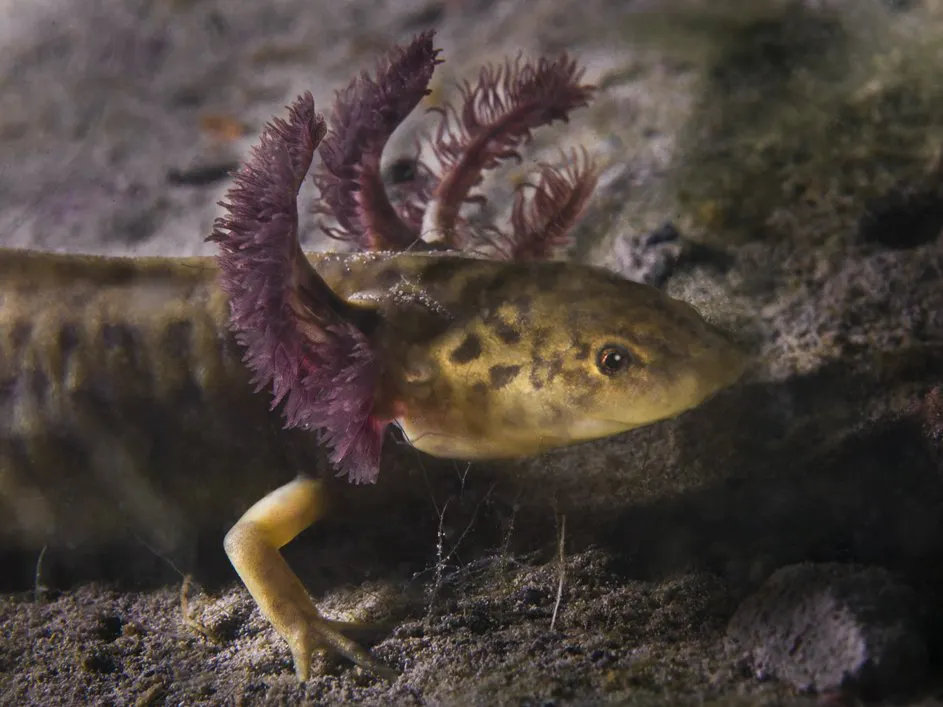## Things used in this project

### Hardware components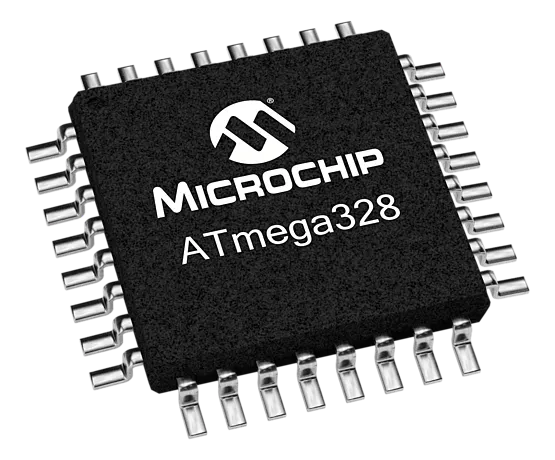Microchip ATmega328
×1
 SparkFun Weather Meters
×1
 Adafruit Mesh-protected Weather-proof Temperature/Humidity Sensor - SHT10
×1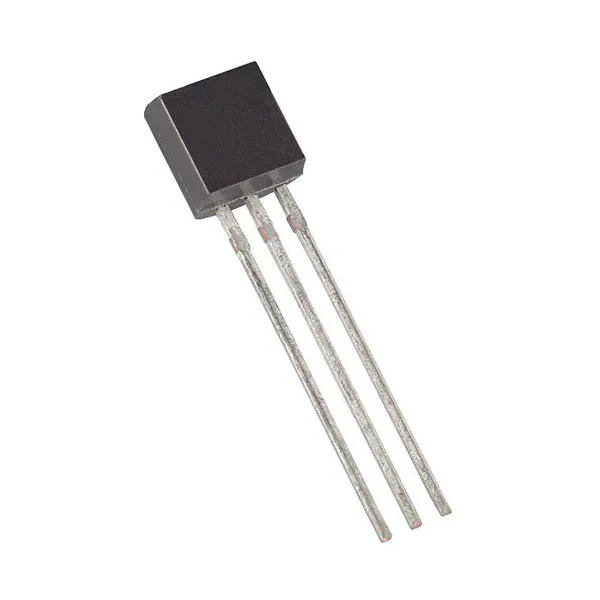Maxim Integrated DS18B20 Programmable Resolution 1-Wire Digital Thermometer
×1
×1
 ICP-10100
×1
 SparkFun pH Sensor
×1
 SparkFun Salinity Sensor
×1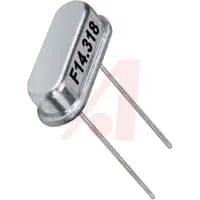16 MHz Crystal
×1
 Seeed Grove connectors
×1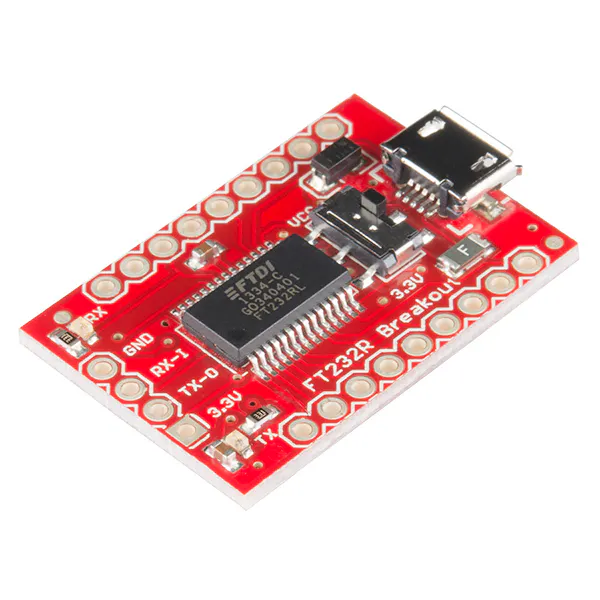SparkFun USB to Serial Breakout - FT232RL
×1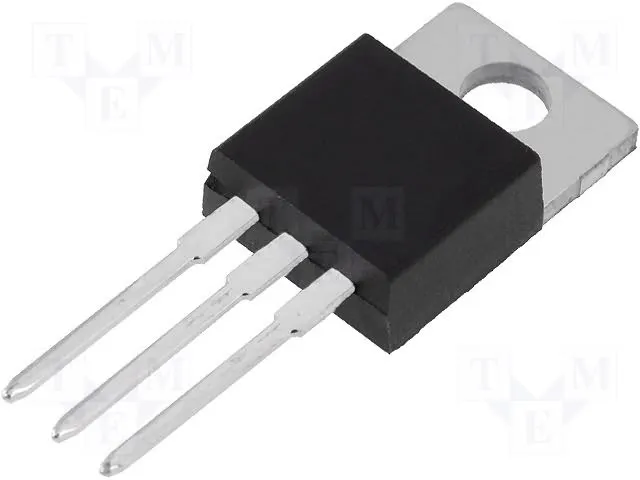Linear Regulator (7805)
×1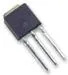Power MOSFET N-Channel
×1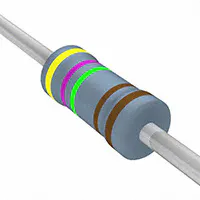Resistor 4.75k ohm
×1Resistor 1k ohm
×1Resistor 10k ohm
×1

### Software apps and online servicesArduino IDE
 Autodesk EagleCircuitMaker by Altium Circuit MakerUbidotsSigfox

### Hand tools and fabrication machines

 Drill
 Ultimaker 2+

## Custom parts and enclosures

3D view

### PCB GERBER FILES

This attachment have the necessary to the production or you own circuit, but if you need one circuit, you can contact us to send you one o help your necessities.

## Schematics

### Schematic

Schematic in pdf.

## Code

### AM2315 Sensor

C/C++
Test to use the sensor
```#include <Wire.h>

// Connect RED of the AM2315 sensor to 5.0V
// Connect BLACK to Ground
// Connect WHITE to i2c clock - on '168/'328 Arduino Uno/Duemilanove/etc thats Analog 5, with a 10 k pull-up resistor
// Connect YELLOW to i2c data - on '168/'328 Arduino Uno/Duemilanove/etc thats Analog 4, with a 10 k pull-up resistor

void setup() {
Serial.begin(9600);
Serial.println("AM2315 Test!");

if (! am2315.begin()) {
while (1);
}
}

void loop() {

delay(1000);
}
```

### DS18B20 Sensor

C/C++
Test to use the sensor
```#include <OneWire.h>
#include <DallasTemperature.h>

// Pin donde se conecta el bus 1-Wire
const int pinDatosDQ = 9;

// Instancia a las clases OneWire y DallasTemperature
OneWire oneWireObjeto(pinDatosDQ);
DallasTemperature sensorDS18B20(&oneWireObjeto);

void setup() {
// Iniciamos la comunicación serie
Serial.begin(9600);
// Iniciamos el bus 1-Wire
sensorDS18B20.begin();
}

void loop() {
// Mandamos comandos para toma de temperatura a los sensores
Serial.println("Mandando comandos a los sensores");
sensorDS18B20.requestTemperatures();

Serial.print("Temperatura sensor 0: ");
Serial.print(sensorDS18B20.getTempCByIndex(0));
Serial.println(" C");
Serial.print("Temperatura sensor 1: ");

delay(1000);
}
```

### SHT10 Sensor

C/C++
Test to use the sensor
```#include <SHT1x.h>

#define dataPin  10
#define clockPin 11
SHT1x sht10(dataPin, clockPin);

void setup()
{
Serial.begin(9600); // Open serial connection to report values to host
Serial.println("Starting up");
}

void loop()
{
float temp_c;
float temp_f;
float humidity;

// Read values from the sensor

// Print the values to the serial port
Serial.print("Temperature: ");
Serial.print(temp_c);
Serial.print("C / ");
Serial.print(" Humidity: ");
Serial.print(humidity);
Serial.println("%");

delay(2000);
}
```

### PH-4502C Sensor

C/C++
Test to use the sensor
```const byte pHpin = A0;// Connect the sensor's Po output to analogue pin 0.
float Po;
void setup()
{
Serial.begin(9600);
}

void loop()
{
Po = (1023 - analogRead(pHpin)) / 73.07; // Read and reverse the analogue input value from the pH sensor then scale 0-14.
Serial.println(Po, 2);// Print the result in the serial monitor.
delay(1000);// Take 1 reading per second.
}
```

### Example to send data to sigfox

C/C++
```#include <SoftwareSerial.h>
SoftwareSerial Sigfox(2, 3); // RX, TX

const int boton=6;
char RespuestaSigfox;

void setup() {
// put your setup code here, to run once:
Serial.begin(9600);
Sigfox.begin(9600);
pinMode(boton, INPUT);
pinMode(7, OUTPUT);

}

void enviar_sigfox(int x)
{
String bufer="AT\$SF=";
Serial.print("Enviar: ");
Serial.println(x);
//convierte el dato a bytes y lo agrega a nuestro mensaje a enviar
String str1;
str1=  String(x, HEX);
bufer+=str1;
//
digitalWrite(7, HIGH);
delay(1000);
enviarcomandoATSigfox("AT");
enviarcomandoATSigfox("AT\$RC");
digitalWrite(7, LOW);
}

void loop() {
// put your main code here, to run repeatedly:
{
enviar_sigfox(60);
delay(2000);
}
}

void enviarcomandoATSigfox(char* comandoAT){
unsigned long x=0;
x = 0;
memset(RespuestaSigfox, '\0',sizeof(RespuestaSigfox));
Sigfox.print(comandoAT);
Sigfox.print("\r\n");
Serial.print(comandoAT);
Serial.print("\r\n");

while(true){
if(Sigfox.available() != 0){
x++;
if (strstr(RespuestaSigfox, "\n") != NULL){
break;
}
}
}
}
```

### Array 12 bits

C/C++
```#define Variable1 3.5
#define Variable2 17.5
#define Variable3 37.6
#define Variable4 161
#define Variable5 187
#define Variable6 255
#define Variable7 100
#define Variable8 5
char s;
int pbit1;
int pbit2;
char bit1;
char bit2;

void setup() {
Serial.begin(9600);
calculo(Variable1);
s=bit1;
s=bit2;
calculo(Variable2);
s=bit1;
s=bit2;
calculo(Variable3);
s=bit1;
s=bit2;
calculo(Variable4);
s=bit1;
s=bit2;
calculo(Variable5);
s=bit1;
s=bit2;
calculo(Variable6);
s=bit1;
s=bit2;
calculo(Variable7);
s=bit1;
s=bit2;
calculo(Variable8);
s=bit1;
s=bit2;
Serial.print("  ");

for(int i=0; i<=15; i++){
Serial.print(s[i]);
Serial.print(" ");}
}

void loop() {
}

void calculo(int variable){
pbit1=variable/16;
if(pbit1==10 ){
bit1='A';}
else if(pbit1==11){
bit1='B';}
else if(pbit1==12){
bit1='C';}
else if(pbit1==13){
bit1='D';}
else if(pbit1==14){
bit1='E';}
else if(pbit1==15){
bit1='F';}
else{bit1=pbit1+'0';}

pbit2=variable-(pbit1*16);

if(pbit2==10 ){
bit2='A';}
else if(pbit2==11){
bit2='B';}
else if(pbit2==12){
bit2='C';}
else if(pbit2==13){
bit1='D';}
else if(pbit2==14){
bit2='E';}
else if(pbit2==15){
bit2='F';}
else{bit2=pbit2+'0';}
Serial.print(bit1);
Serial.print(bit2);
}
```

### Send to Ubidots

C/C++
This code send hexadecimal data to sigfox plataform and convert this data to decimal values and then send the same data to Ubidots
```#define Variable1 3
#define Variable2 17
#define Variable3 37
#define Variable4 161
#define Variable5 187
#define Variable6 255
#define Variable7 100
#define Variable8 5

String s="0000000000000000";
int pbit1;
int pbit2;
char bit1;
char bit2;

#include <SoftwareSerial.h>
SoftwareSerial Sigfox(2, 3); // RX, TX

const int boton=6;
char RespuestaSigfox;

void setup() {
Serial.begin(9600);
Sigfox.begin(9600);
pinMode(boton, INPUT);
pinMode(7, OUTPUT);
calculo(Variable1);
s=bit1;
s=bit2;
calculo(Variable2);
s=bit1;
s=bit2;
calculo(Variable3);
s=bit1;
s=bit2;
calculo(Variable4);
s=bit1;
s=bit2;
calculo(Variable5);
s=bit1;
s=bit2;
calculo(Variable6);
s=bit1;
s=bit2;
calculo(Variable7);
s=bit1;
s=bit2;
calculo(Variable8);
s=bit1;
s=bit2;

Serial.print(" ");
Serial.print(s);

pinMode(boton, INPUT);
pinMode(7, OUTPUT);
}

void enviar_sigfox(int x)
{
String bufer="AT\$SF=";
Serial.println("Enviar: ");
Serial.println(s);
//convierte el dato a bytes y lo agrega a nuestro mensaje a enviar
String str1;
// str1=  String(x, HEX);
bufer+=s;
//
digitalWrite(7, HIGH);
delay(1000);
enviarcomandoATSigfox("AT");
enviarcomandoATSigfox("AT\$RC");
digitalWrite(7, LOW);
}

void loop() {
// put your main code here, to run repeatedly:
{
enviar_sigfox(60);
delay(2000);
}
}

void calculo(int variable){
pbit1=variable/16;
if(pbit1==10 ){
bit1='a';}
else if(pbit1==11){
bit1='b';}
else if(pbit1==12){
bit1='c';}
else if(pbit1==13){
bit1='d';}
else if(pbit1==14){
bit1='e';}
else if(pbit1==15){
bit1='f';}
else{bit1=pbit1+'0';}

pbit2=variable-(pbit1*16);

if(pbit2==10 ){
bit2='a';}
else if(pbit2==11){
bit2='b';}
else if(pbit2==12){
bit2='c';}
else if(pbit2==13){
bit1='d';}
else if(pbit2==14){
bit2='e';}
else if(pbit2==15){
bit2='f';}
else{bit2=pbit2+'0';}
Serial.print(bit1);
Serial.print(bit2);
}

void enviarcomandoATSigfox(char* comandoAT){
unsigned long x=0;
x = 0;
memset(RespuestaSigfox, '\0',sizeof(RespuestaSigfox));
Sigfox.print(comandoAT);
Sigfox.print("\r\n");
Serial.print(comandoAT);
Serial.print("\r\n");

while(true){
if(Sigfox.available() != 0){
x++;
if (strstr(RespuestaSigfox, "\n") != NULL){
break;
}
}
}
}
```

## Credits

### Mario De Los Santos

1 project • 1 follower
Having fun with electronics and programming